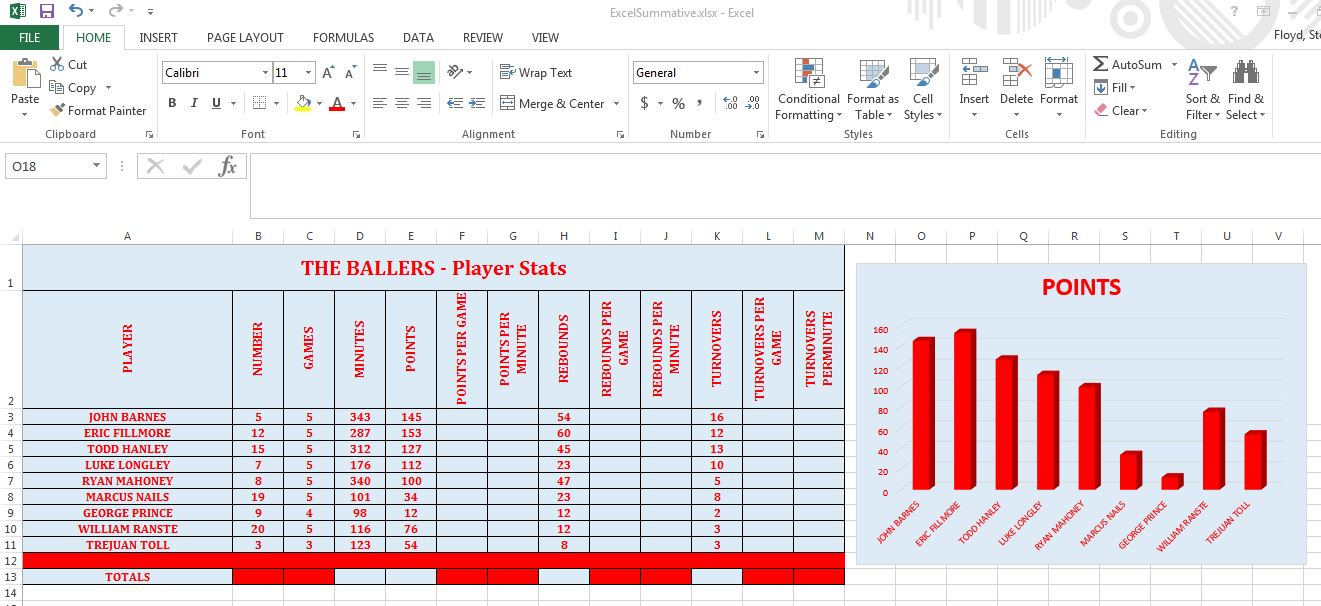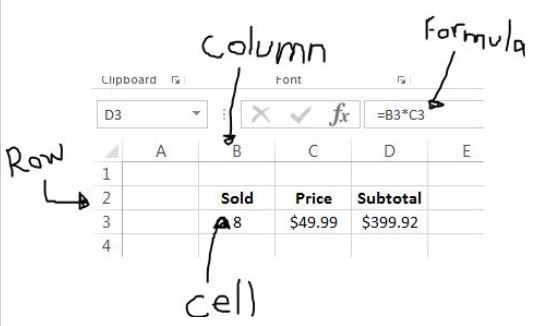# MSExcel - The Basics

MSExcel is software that allows you to create spreadsheets.

Spreadsheets hold data, values, statistics and formulas.
Spreadsheet software can also allow us to chart the data, which is pretty useful for presenting.

This is a sample of a spreadhseet that keeps track of statistics for a basketball team:Some important terms that you need to know right away include:

Workbook vs Worksheet:
A workbook is your whole spreadsheet file, it is saved under one name.
A worksheet is one sheet inside the workbook.
So a workbook might have a whole bunch of worksheets associated with it.
You can see the different worksheets on the tabs at the bottom.

Row vs Column:
A row goes horizontally across a page. In excel they are numbered 1, 2, 3, 4, etc...
A column goes doewn the page, they are labelled A, B, C, D, E...
A cell is an intersection of a row and a column. So cell A4 would be column A, row 4.

Formulas:
Formulas are super useful in spreadsheets.
Formulas allow the computer to do all the calculations for you, which is awesome!
If you have a spreadsheet that includes the number of tennis rackets you sold (like 8) and the price of the tennis racket (like \$49.99) then you can make a formula that will multiply the amount sold times the price.The formula above multiplies cell B3 by C3 and stores the result in D3.
If a user goes and changes the number sold, then the formula will stay the same, but the result (\$399.92) will change based on the new data.